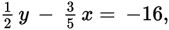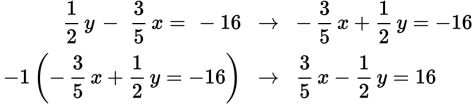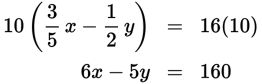# SAT Math Multiple Choice Question 344: Answer and Explanation

### Test Information

Question: 344

14. Ifwhat is the value of 6x - 5y?

• A. 32
• B. 80
• C. 96
• D. 160

Explanation:

D

Difficulty: Hard

Category: Heart of Algebra / Linear Equations

Strategic Advice: There is only one equation given, and it has two variables. This means that you don't have enough information to solve for either variable. Instead, look for the relationship between the left side of the equation and the other expression that you are trying to find.

Getting to the Answer: The expression you are trying to find (6x - 5y) has the x-term first and then the y-term, so start by reversing the order of the terms on the left side of the given equation. Also, notice that the x term in 6x - 5y is not negative, so multiply the equation by -1.Finally, there are no fractions in the desired expression, so clear the fractions by multiplying both sides of the equation by 10. This yields the expression that you are looking for, so no further work is required-just read the value on the right-hand side of the equation, which is 160.